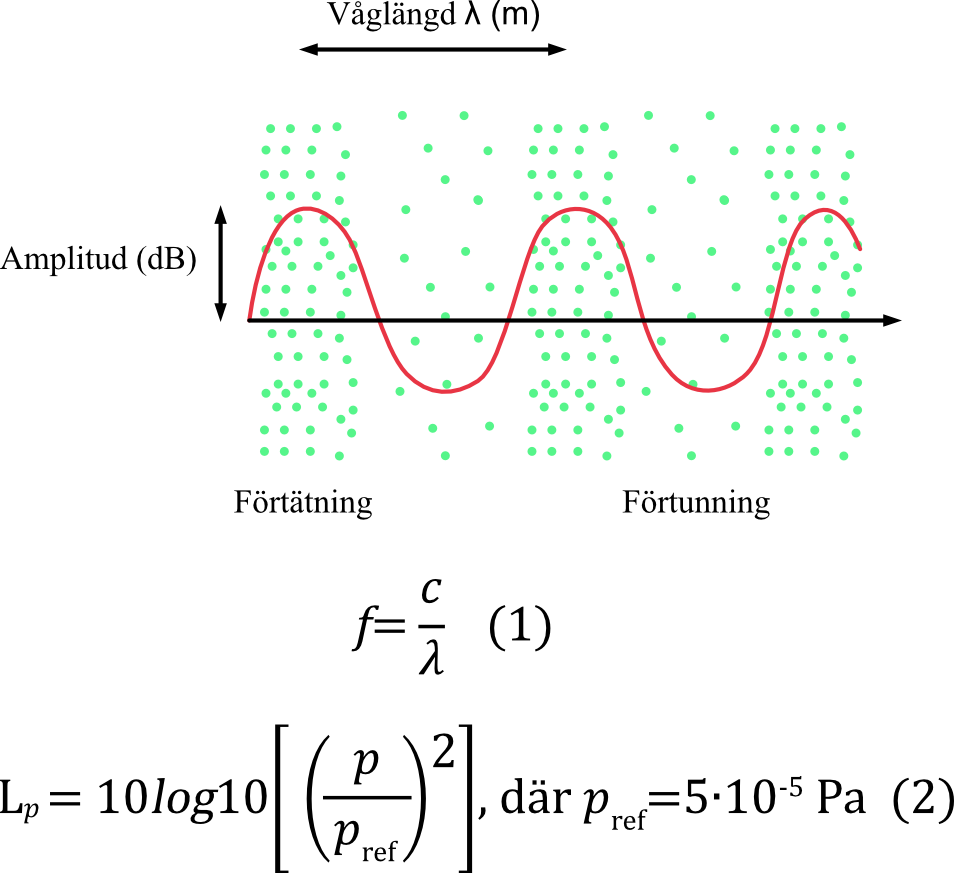# Sound and its phenomena

Sound is generated by air molecules starting to oscillate in response to vibrations. The air molecules collide with each other, causing them to concentrate (compression) and spread out (rarefaction). The air molecules do not travel far, whereas the compressions and rarefactions create the wave motions. The rarefactions and compressions propagate in all directions from the acoustic source.

The distance between two waves (compression to compression) is defined as wavelength (λ). The sound frequency (f) is calculated as the speed of the sound (c) divided by the wavelength (equation 1), c in air is 340 m/s. If the wavelength is 10 m, the frequency will be 34 Hz. The human ear can detect sounds from 20 to 20,000 Hz. The frequency determines the pitch of a sound.

If turning up the volume when listening to music, the volume increases and the sound waves get higher. The height of the sound waves is called amplitude, sound pressure. The sound pressure (Pa) is very variable, therefore expressed in sound levels (Lp) in dB, with the calculation performed using equation 2. The decibel is a logarithmic unit used to make it easier to understand.